Скачать презентацию Boosted Sampling Approximation Algorithms for Stochastic Problems Martin

ab75669d7ee0166fd519673b33a4aa79.ppt

• Количество слайдов: 30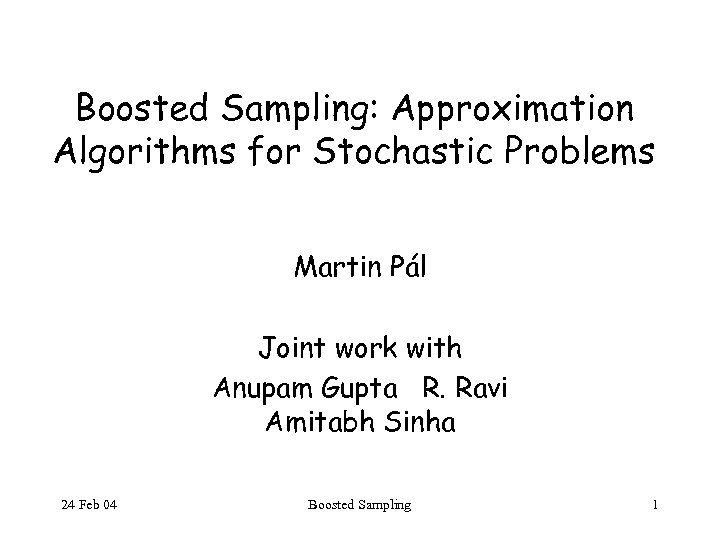Boosted Sampling: Approximation Algorithms for Stochastic Problems Martin Pál Joint work with Anupam Gupta R. Ravi Amitabh Sinha 24 Feb 04 Boosted Sampling 1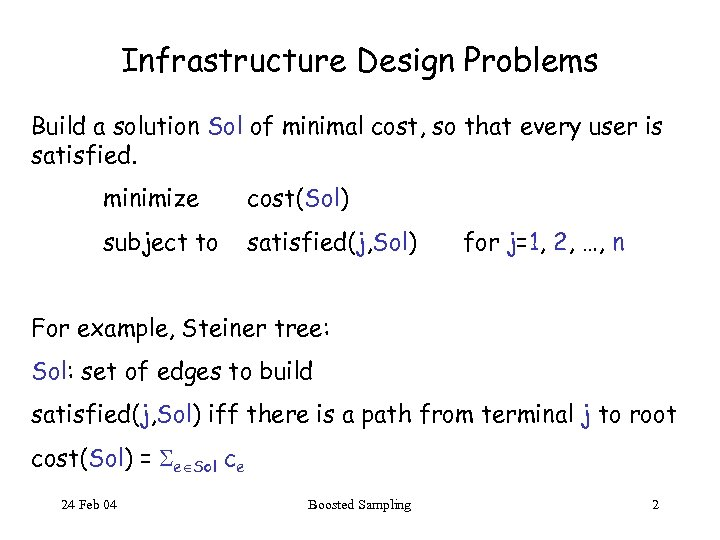Infrastructure Design Problems Build a solution Sol of minimal cost, so that every user is satisfied. minimize cost(Sol) subject to satisfied(j, Sol) for j=1, 2, …, n For example, Steiner tree: Sol: set of edges to build satisfied(j, Sol) iff there is a path from terminal j to root cost(Sol) = e Sol ce 24 Feb 04 Boosted Sampling 2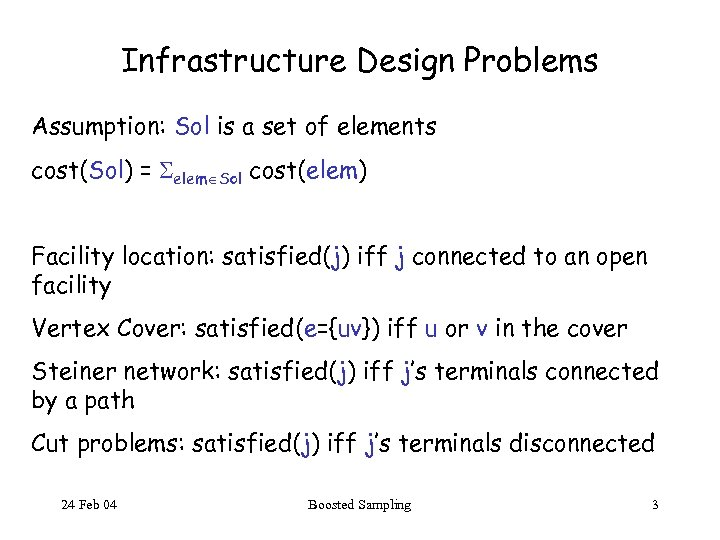Infrastructure Design Problems Assumption: Sol is a set of elements cost(Sol) = elem Sol cost(elem) Facility location: satisfied(j) iff j connected to an open facility Vertex Cover: satisfied(e={uv}) iff u or v in the cover Steiner network: satisfied(j) iff j’s terminals connected by a path Cut problems: satisfied(j) iff j’s terminals disconnected 24 Feb 04 Boosted Sampling 3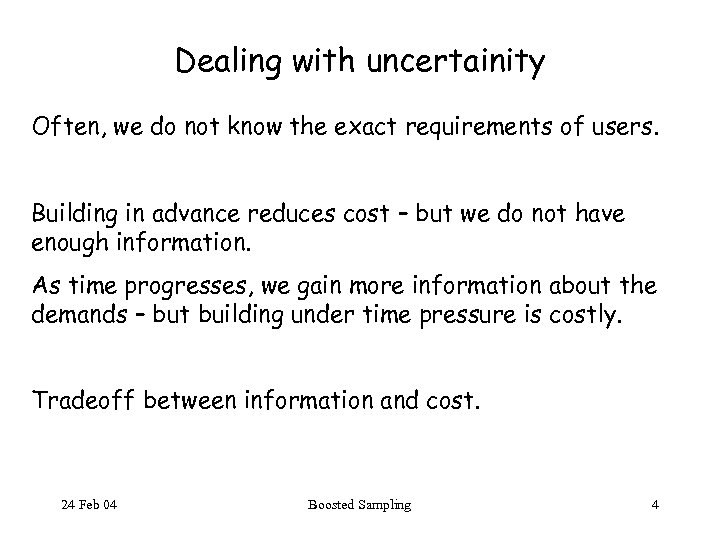Dealing with uncertainity Often, we do not know the exact requirements of users. Building in advance reduces cost – but we do not have enough information. As time progresses, we gain more information about the demands – but building under time pressure is costly. Tradeoff between information and cost. 24 Feb 04 Boosted Sampling 4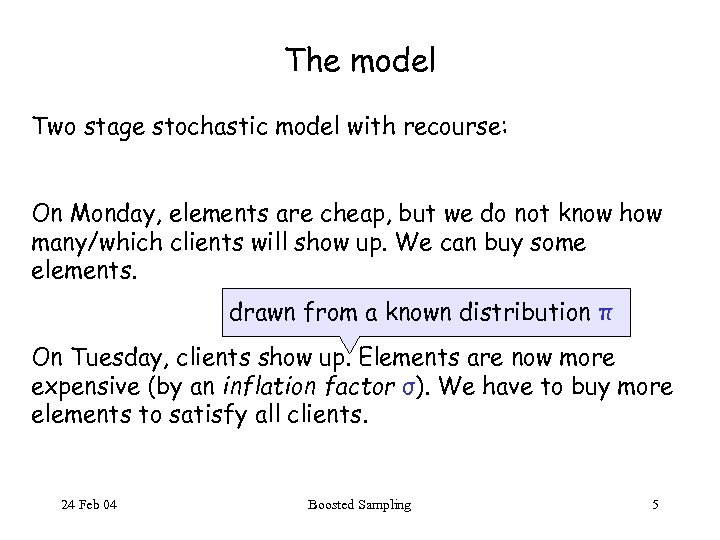The model Two stage stochastic model with recourse: On Monday, elements are cheap, but we do not know how many/which clients will show up. We can buy some elements. drawn from a known distribution π On Tuesday, clients show up. Elements are now more expensive (by an inflation factor σ). We have to buy more elements to satisfy all clients. 24 Feb 04 Boosted Sampling 5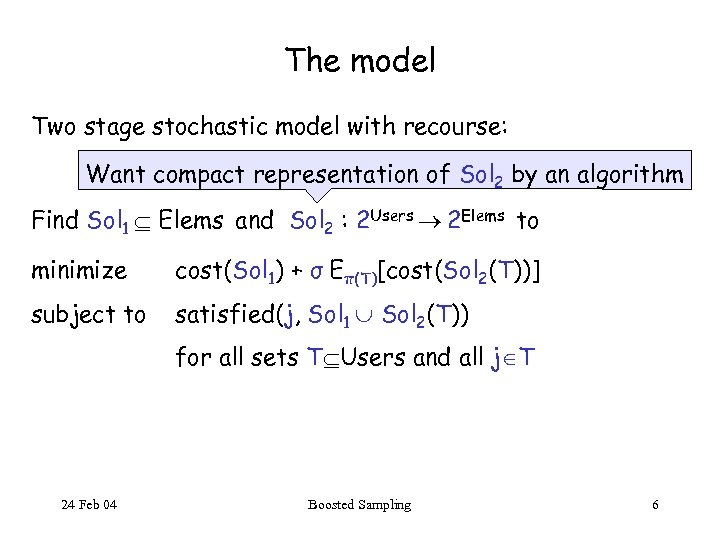The model Two stage stochastic model with recourse: Want compact representation of Sol 2 by an algorithm Find Sol 1 Elems and Sol 2 : 2 Users 2 Elems to minimize cost(Sol 1) + σ Eπ(T)[cost(Sol 2(T))] subject to satisfied(j, Sol 1 Sol 2(T)) for all sets T Users and all j T 24 Feb 04 Boosted Sampling 6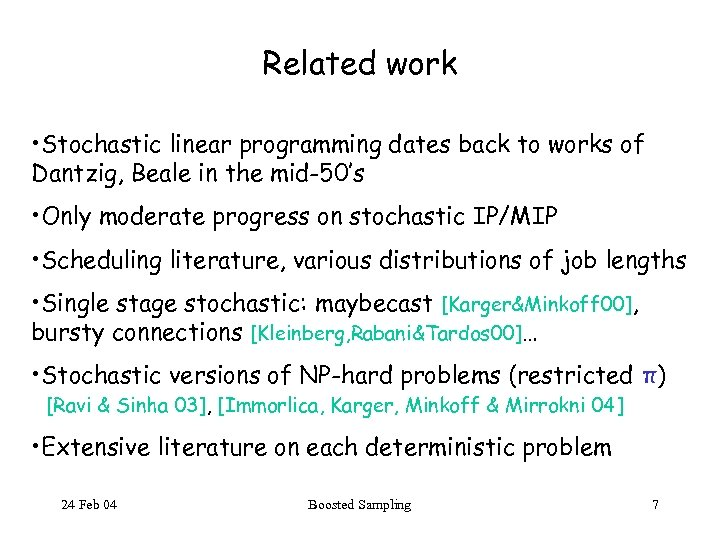Related work • Stochastic linear programming dates back to works of Dantzig, Beale in the mid-50’s • Only moderate progress on stochastic IP/MIP • Scheduling literature, various distributions of job lengths • Single stage stochastic: maybecast [Karger&Minkoff 00], bursty connections [Kleinberg, Rabani&Tardos 00]… • Stochastic versions of NP-hard problems (restricted π) [Ravi & Sinha 03], [Immorlica, Karger, Minkoff & Mirrokni 04] • Extensive literature on each deterministic problem 24 Feb 04 Boosted Sampling 7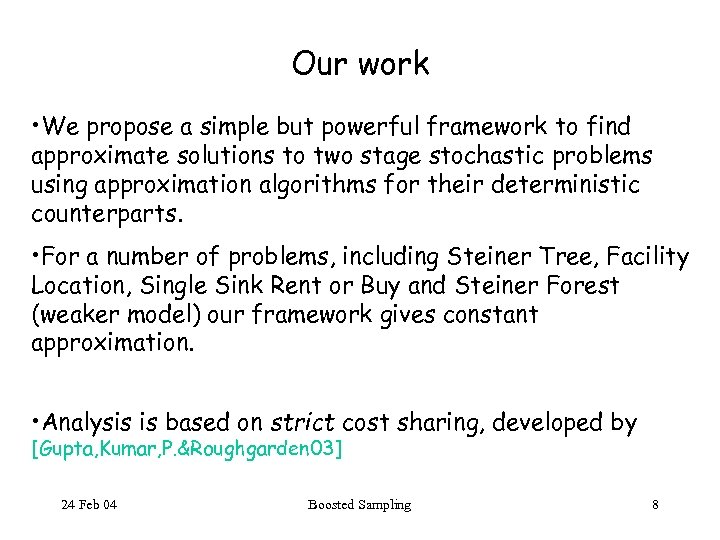Our work • We propose a simple but powerful framework to find approximate solutions to two stage stochastic problems using approximation algorithms for their deterministic counterparts. • For a number of problems, including Steiner Tree, Facility Location, Single Sink Rent or Buy and Steiner Forest (weaker model) our framework gives constant approximation. • Analysis is based on strict cost sharing, developed by [Gupta, Kumar, P. &Roughgarden 03] 24 Feb 04 Boosted Sampling 8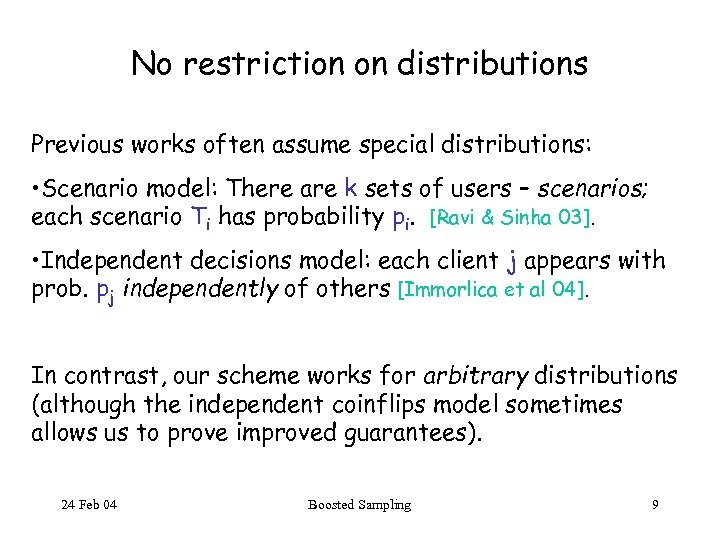No restriction on distributions Previous works often assume special distributions: • Scenario model: There are k sets of users – scenarios; each scenario Ti has probability pi. [Ravi & Sinha 03]. • Independent decisions model: each client j appears with prob. pj independently of others [Immorlica et al 04]. In contrast, our scheme works for arbitrary distributions (although the independent coinflips model sometimes allows us to prove improved guarantees). 24 Feb 04 Boosted Sampling 9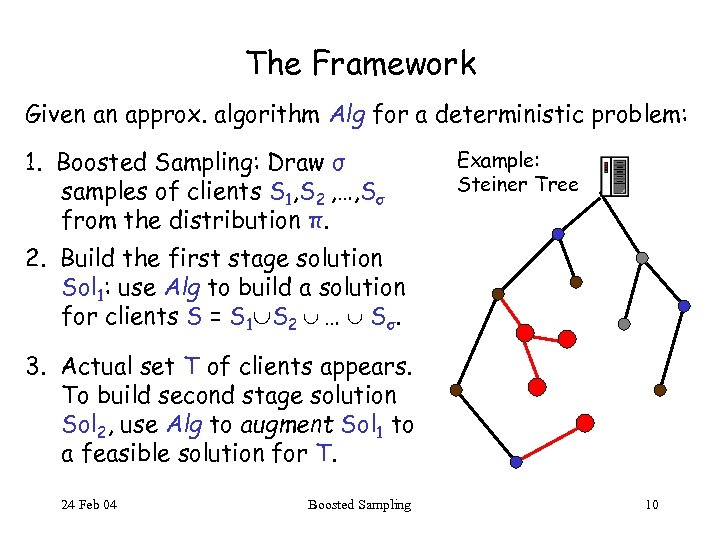The Framework Given an approx. algorithm Alg for a deterministic problem: 1. Boosted Sampling: Draw σ samples of clients S 1, S 2 , …, Sσ from the distribution π. Example: Steiner Tree 2. Build the first stage solution Sol 1: use Alg to build a solution for clients S = S 1 S 2 … Sσ. 3. Actual set T of clients appears. To build second stage solution Sol 2, use Alg to augment Sol 1 to a feasible solution for T. 24 Feb 04 Boosted Sampling 10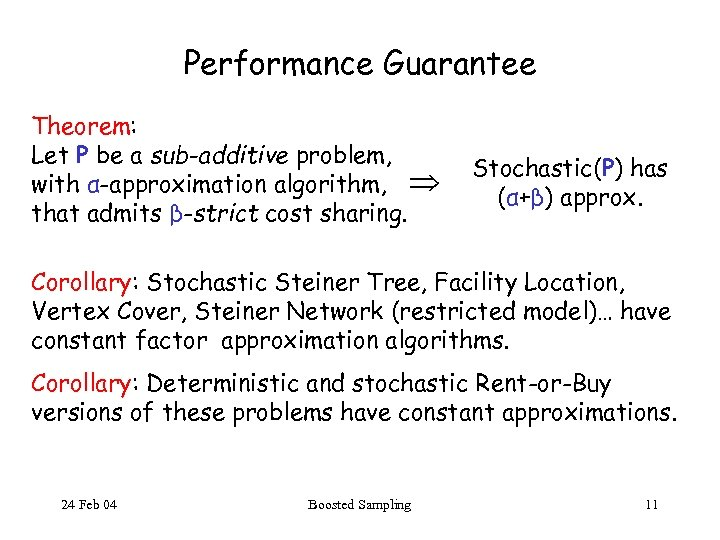Performance Guarantee Theorem: Let P be a sub-additive problem, with α-approximation algorithm, that admits β-strict cost sharing. Stochastic(P) has (α+β) approx. Corollary: Stochastic Steiner Tree, Facility Location, Vertex Cover, Steiner Network (restricted model)… have constant factor approximation algorithms. Corollary: Deterministic and stochastic Rent-or-Buy versions of these problems have constant approximations. 24 Feb 04 Boosted Sampling 11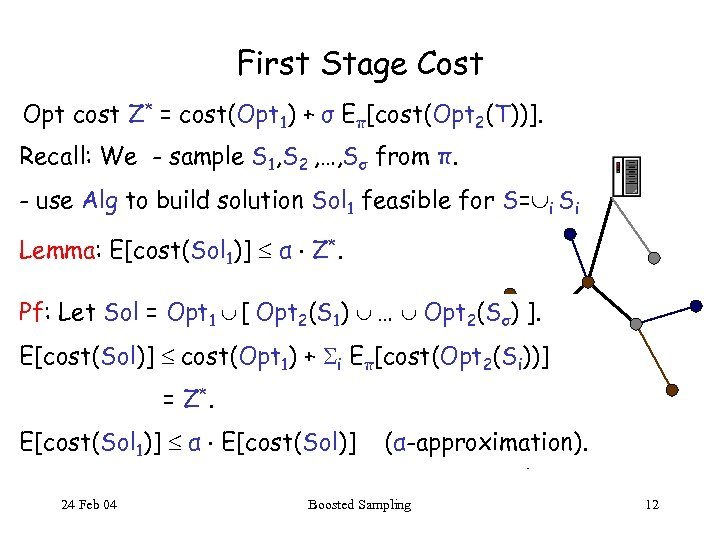First Stage Cost Opt cost Z* = cost(Opt 1) + σ Eπ[cost(Opt 2(T))]. Recall: We - sample S 1, S 2 , …, Sσ from π. - use Alg to build solution Sol 1 feasible for S= i Si Lemma: E[cost(Sol 1)] α Z*. Pf: Let Sol = Opt 1 [ Opt 2(S 1) … Opt 2(Sσ) ]. E[cost(Sol)] cost(Opt 1) + i Eπ[cost(Opt 2(Si))] = Z*. E[cost(Sol 1)] α E[cost(Sol)] 24 Feb 04 (α-approximation). Boosted Sampling 12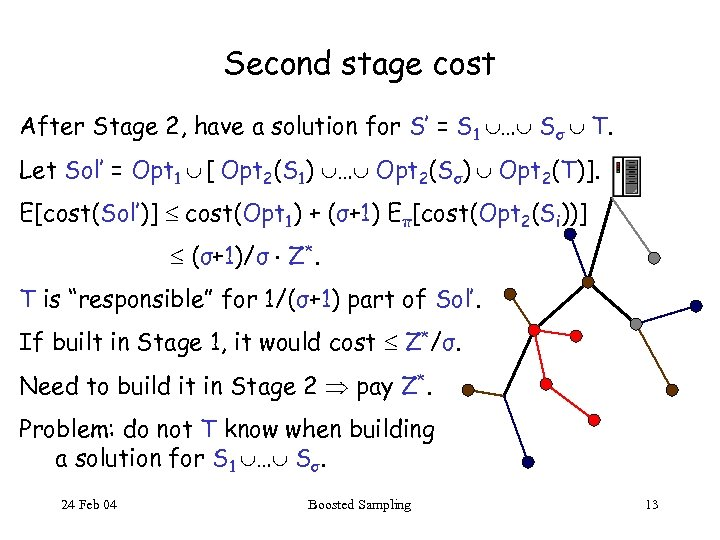Second stage cost After Stage 2, have a solution for S’ = S 1 … Sσ T. Let Sol’ = Opt 1 [ Opt 2(S 1) … Opt 2(Sσ) Opt 2(T)]. E[cost(Sol’)] cost(Opt 1) + (σ+1) Eπ[cost(Opt 2(Si))] (σ+1)/σ Z*. T is “responsible” for 1/(σ+1) part of Sol’. If built in Stage 1, it would cost Z*/σ. Need to build it in Stage 2 pay Z*. Problem: do not T know when building a solution for S 1 … Sσ. 24 Feb 04 Boosted Sampling 13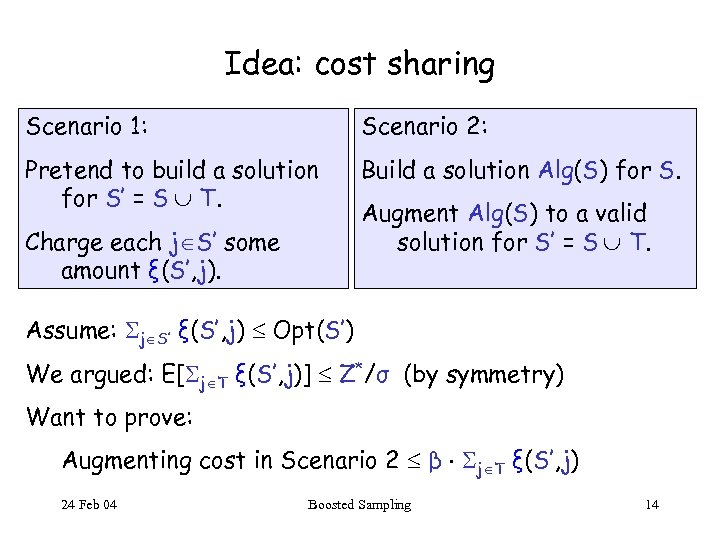Idea: cost sharing Scenario 1: Scenario 2: Pretend to build a solution for S’ = S T. Build a solution Alg(S) for S. Charge each j S’ some amount ξ(S’, j). Augment Alg(S) to a valid solution for S’ = S T. Assume: j S’ ξ(S’, j) Opt(S’) We argued: E[ j T ξ(S’, j)] Z*/σ (by symmetry) Want to prove: Augmenting cost in Scenario 2 β j T ξ(S’, j) 24 Feb 04 Boosted Sampling 14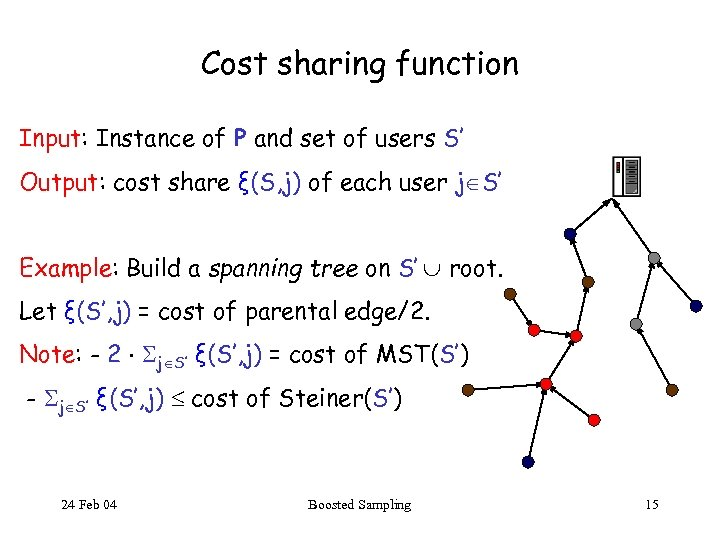Cost sharing function Input: Instance of P and set of users S’ Output: cost share ξ(S, j) of each user j S’ Example: Build a spanning tree on S’ root. Let ξ(S’, j) = cost of parental edge/2. Note: - 2 j S’ ξ(S’, j) = cost of MST(S’) - j S’ ξ(S’, j) cost of Steiner(S’) 24 Feb 04 Boosted Sampling 15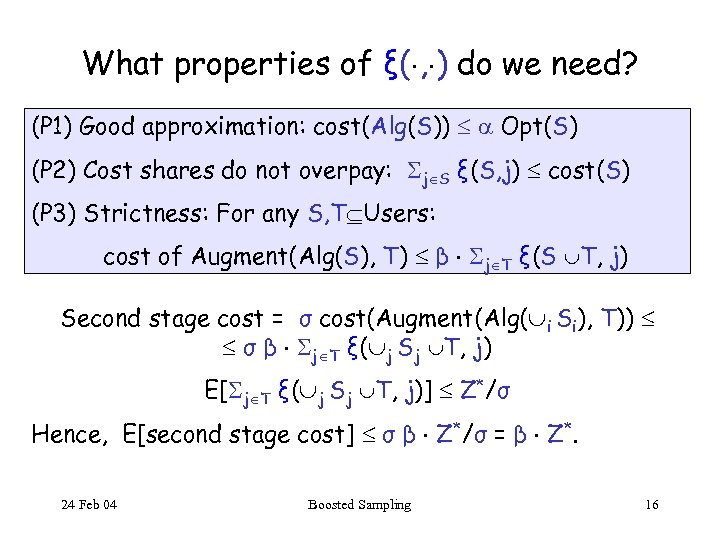What properties of ξ( , ) do we need? (P 1) Good approximation: cost(Alg(S)) Opt(S) (P 2) Cost shares do not overpay: j S ξ(S, j) cost(S) (P 3) Strictness: For any S, T Users: cost of Augment(Alg(S), T) β j T ξ(S T, j) Second stage cost = σ cost(Augment(Alg( i Si), T)) σ β j T ξ( j Sj T, j) E[ j T ξ( j Sj T, j)] Z*/σ Hence, E[second stage cost] σ β Z*/σ = β Z*. 24 Feb 04 Boosted Sampling 16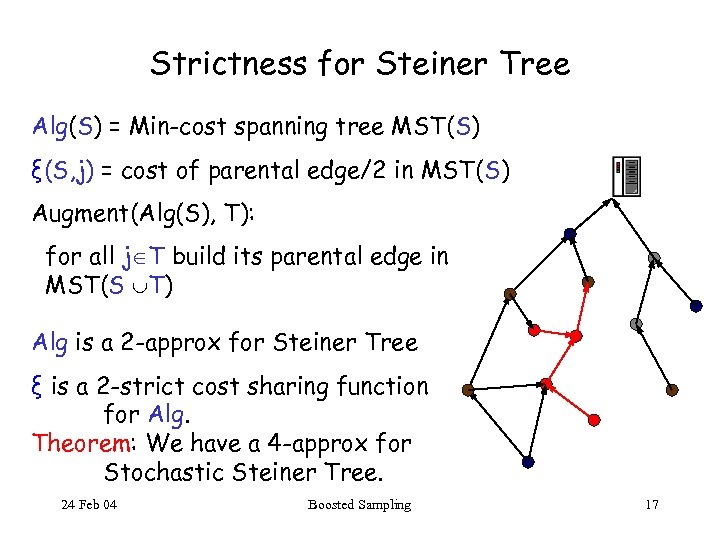Strictness for Steiner Tree Alg(S) = Min-cost spanning tree MST(S) ξ(S, j) = cost of parental edge/2 in MST(S) Augment(Alg(S), T): for all j T build its parental edge in MST(S T) Alg is a 2 -approx for Steiner Tree ξ is a 2 -strict cost sharing function for Alg. Theorem: We have a 4 -approx for Stochastic Steiner Tree. 24 Feb 04 Boosted Sampling 17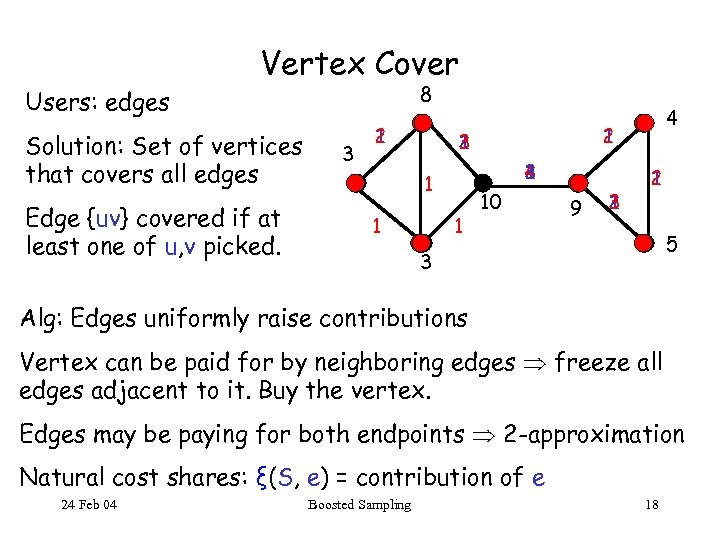Vertex Cover 8 Users: edges Solution: Set of vertices that covers all edges Edge {uv} covered if at least one of u, v picked. 3 2 1 3 4 2 1 1 4 10 9 3 2 1 5 3 Alg: Edges uniformly raise contributions Vertex can be paid for by neighboring edges freeze all edges adjacent to it. Buy the vertex. Edges may be paying for both endpoints 2 -approximation Natural cost shares: ξ(S, e) = contribution of e 24 Feb 04 Boosted Sampling 18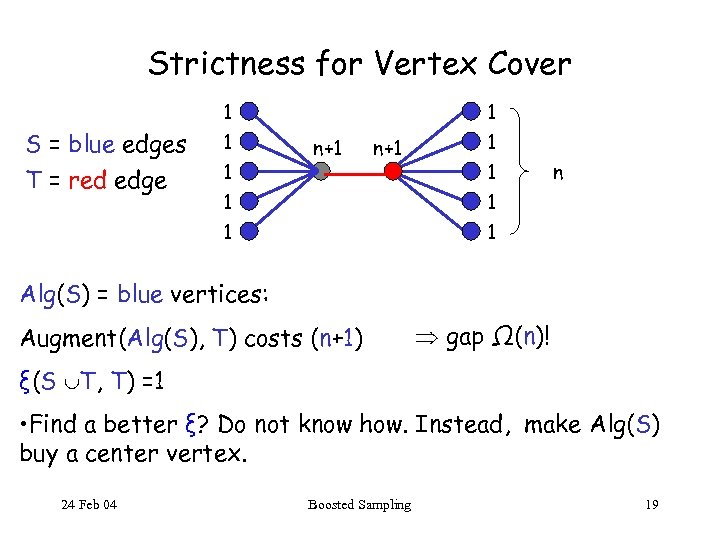Strictness for Vertex Cover 1 S = blue edges T = red edge 1 1 1 n+1 1 1 n Alg(S) = blue vertices: Augment(Alg(S), T) costs (n+1) gap Ω(n)! ξ(S T, T) =1 • Find a better ξ? Do not know how. Instead, make Alg(S) buy a center vertex. 24 Feb 04 Boosted Sampling 19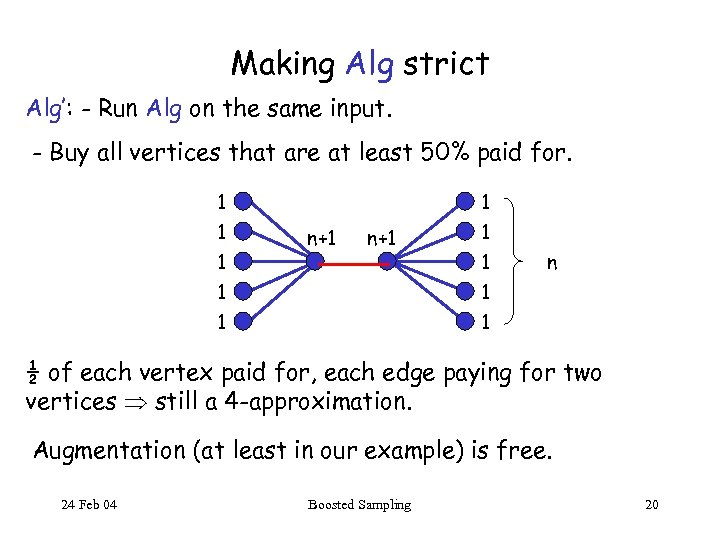Making Alg strict Alg’: - Run Alg on the same input. - Buy all vertices that are at least 50% paid for. 1 1 1 n+1 1 1 n 1 1 ½ of each vertex paid for, each edge paying for two vertices still a 4 -approximation. Augmentation (at least in our example) is free. 24 Feb 04 Boosted Sampling 20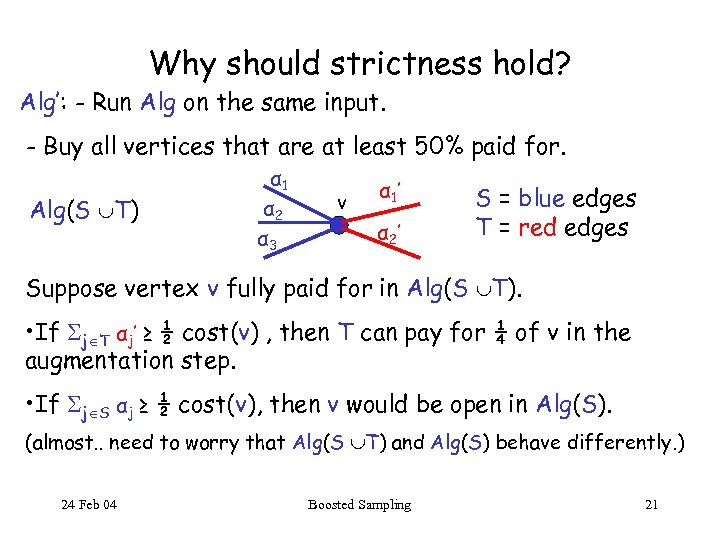Why should strictness hold? Alg’: - Run Alg on the same input. - Buy all vertices that are at least 50% paid for. Alg(S T) α 1 α 2 α 3 v α 1 ’ α 2’ S = blue edges T = red edges Suppose vertex v fully paid for in Alg(S T). • If j T αj’ ≥ ½ cost(v) , then T can pay for ¼ of v in the augmentation step. • If j S αj ≥ ½ cost(v), then v would be open in Alg(S). (almost. . need to worry that Alg(S T) and Alg(S) behave differently. ) 24 Feb 04 Boosted Sampling 21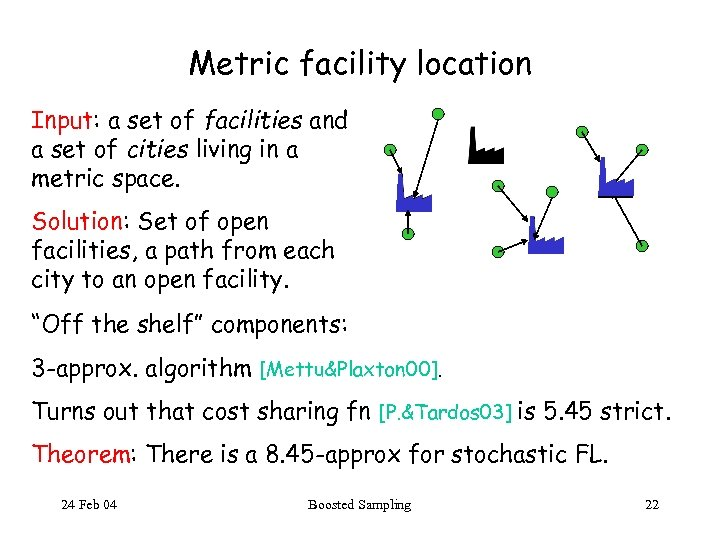Metric facility location Input: a set of facilities and a set of cities living in a metric space. Solution: Set of open facilities, a path from each city to an open facility. “Off the shelf” components: 3 -approx. algorithm [Mettu&Plaxton 00]. Turns out that cost sharing fn [P. &Tardos 03] is 5. 45 strict. Theorem: There is a 8. 45 -approx for stochastic FL. 24 Feb 04 Boosted Sampling 22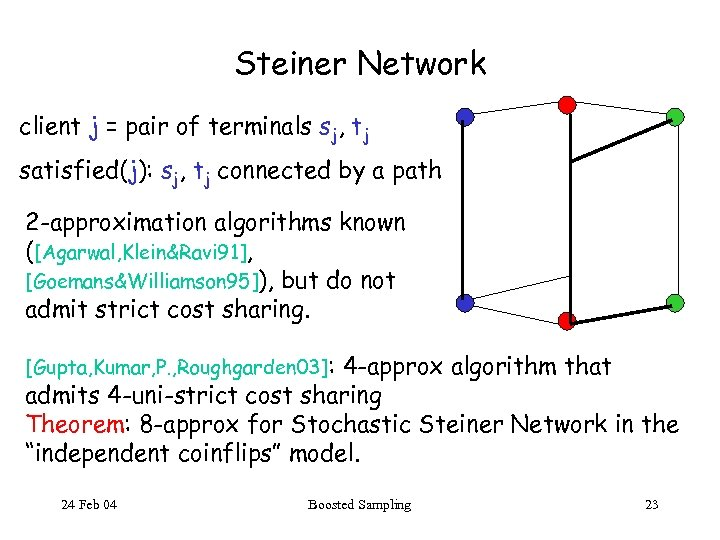Steiner Network client j = pair of terminals sj, tj satisfied(j): sj, tj connected by a path 2 -approximation algorithms known ([Agarwal, Klein&Ravi 91], [Goemans&Williamson 95]), but do not admit strict cost sharing. [Gupta, Kumar, P. , Roughgarden 03]: 4 -approx algorithm that admits 4 -uni-strict cost sharing Theorem: 8 -approx for Stochastic Steiner Network in the “independent coinflips” model. 24 Feb 04 Boosted Sampling 23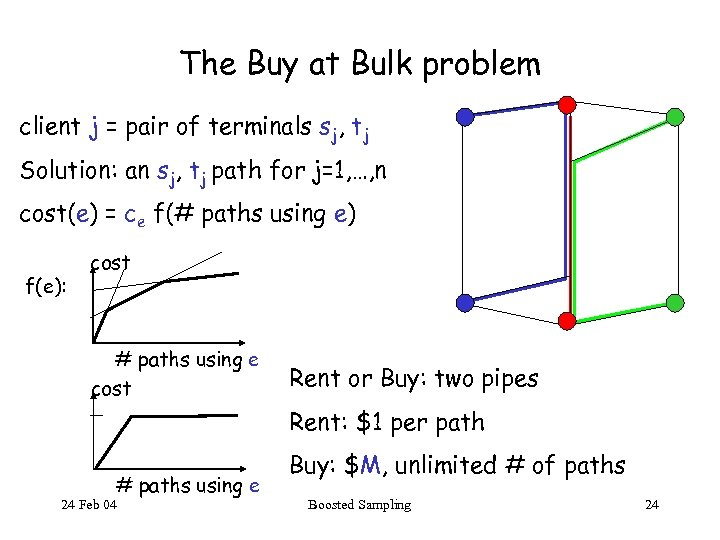The Buy at Bulk problem client j = pair of terminals sj, tj Solution: an sj, tj path for j=1, …, n cost(e) = ce f(# paths using e) f(e): cost # paths using e cost Rent or Buy: two pipes Rent: \$1 per path # paths using e 24 Feb 04 Buy: \$M, unlimited # of paths Boosted Sampling 24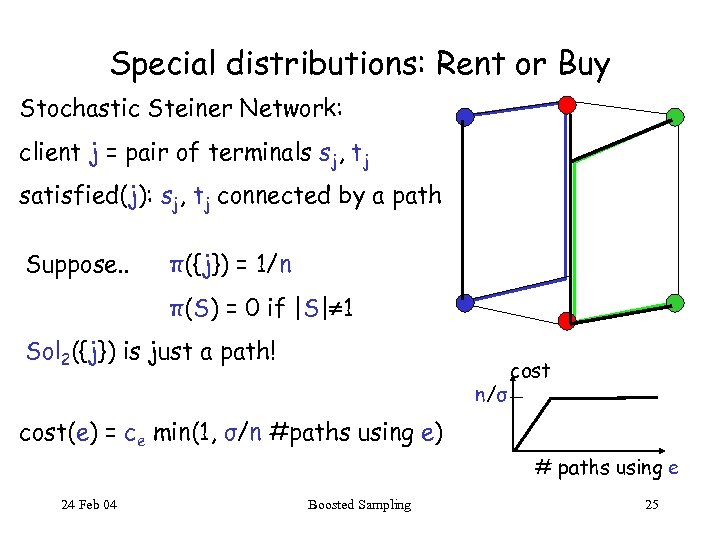Special distributions: Rent or Buy Stochastic Steiner Network: client j = pair of terminals sj, tj satisfied(j): sj, tj connected by a path Suppose. . π({j}) = 1/n π(S) = 0 if |S| 1 Sol 2({j}) is just a path! n/σ cost(e) = ce min(1, σ/n #paths using e) # paths using e 24 Feb 04 Boosted Sampling 25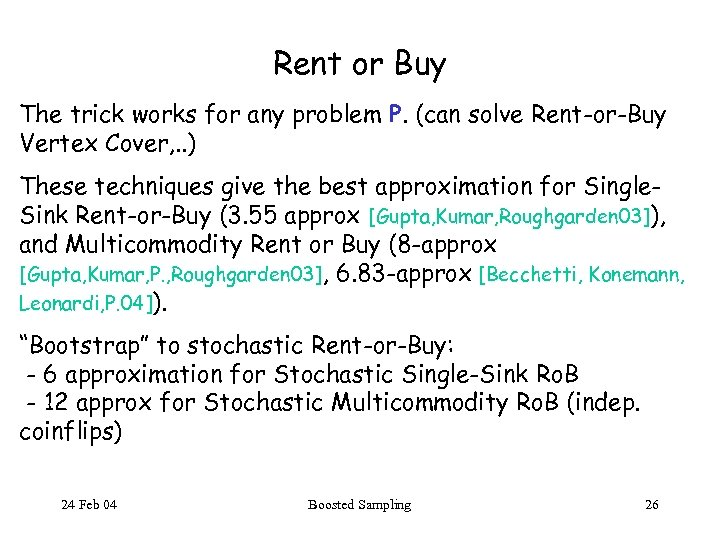Rent or Buy The trick works for any problem P. (can solve Rent-or-Buy Vertex Cover, . . ) These techniques give the best approximation for Single. Sink Rent-or-Buy (3. 55 approx [Gupta, Kumar, Roughgarden 03]), and Multicommodity Rent or Buy (8 -approx [Gupta, Kumar, P. , Roughgarden 03], 6. 83 -approx [Becchetti, Konemann, Leonardi, P. 04]). “Bootstrap” to stochastic Rent-or-Buy: - 6 approximation for Stochastic Single-Sink Ro. B - 12 approx for Stochastic Multicommodity Ro. B (indep. coinflips) 24 Feb 04 Boosted Sampling 26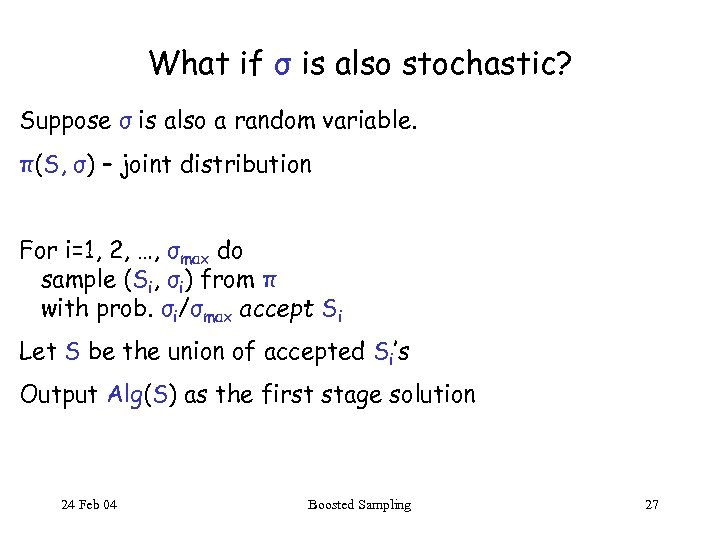What if σ is also stochastic? Suppose σ is also a random variable. π(S, σ) – joint distribution For i=1, 2, …, σmax do sample (Si, σi) from π with prob. σi/σmax accept Si Let S be the union of accepted Si’s Output Alg(S) as the first stage solution 24 Feb 04 Boosted Sampling 27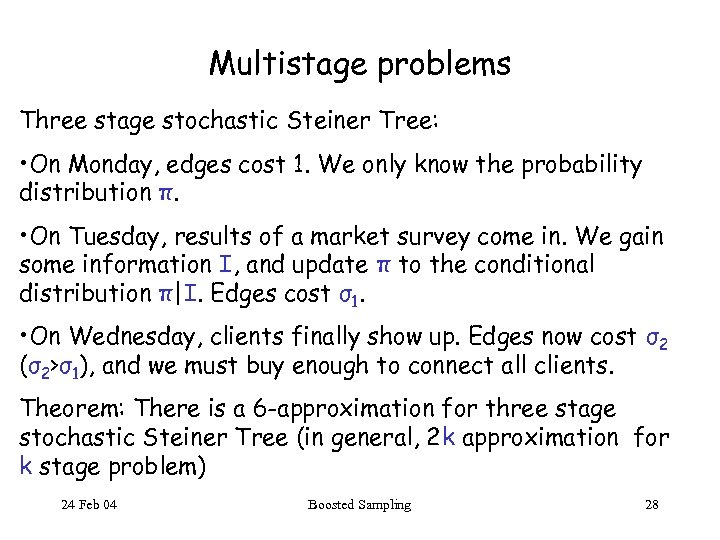Multistage problems Three stage stochastic Steiner Tree: • On Monday, edges cost 1. We only know the probability distribution π. • On Tuesday, results of a market survey come in. We gain some information I, and update π to the conditional distribution π|I. Edges cost σ1. • On Wednesday, clients finally show up. Edges now cost σ2 (σ2>σ1), and we must buy enough to connect all clients. Theorem: There is a 6 -approximation for three stage stochastic Steiner Tree (in general, 2 k approximation for k stage problem) 24 Feb 04 Boosted Sampling 28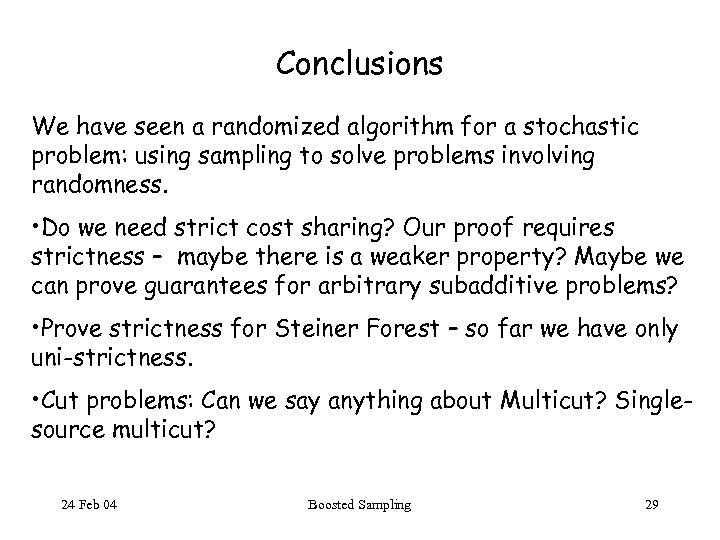Conclusions We have seen a randomized algorithm for a stochastic problem: using sampling to solve problems involving randomness. • Do we need strict cost sharing? Our proof requires strictness – maybe there is a weaker property? Maybe we can prove guarantees for arbitrary subadditive problems? • Prove strictness for Steiner Forest – so far we have only uni-strictness. • Cut problems: Can we say anything about Multicut? Singlesource multicut? 24 Feb 04 Boosted Sampling 29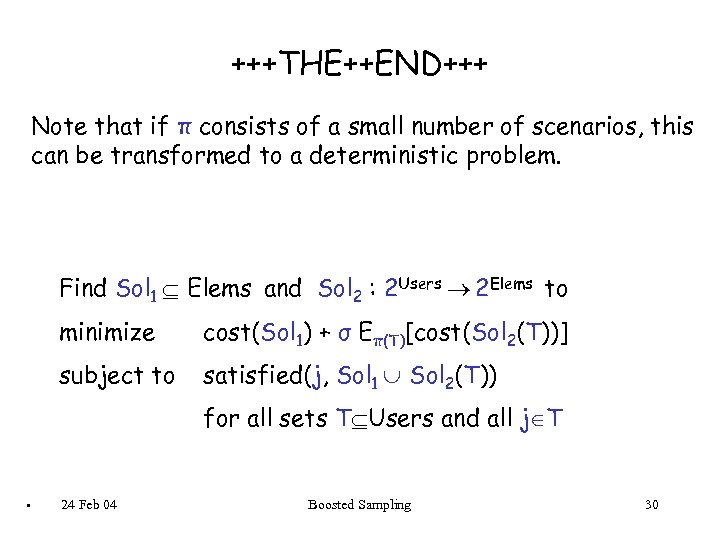+++THE++END+++ Note that if π consists of a small number of scenarios, this can be transformed to a deterministic problem. Find Sol 1 Elems and Sol 2 : 2 Users 2 Elems to minimize cost(Sol 1) + σ Eπ(T)[cost(Sol 2(T))] subject to satisfied(j, Sol 1 Sol 2(T)) for all sets T Users and all j T . 24 Feb 04 Boosted Sampling 30